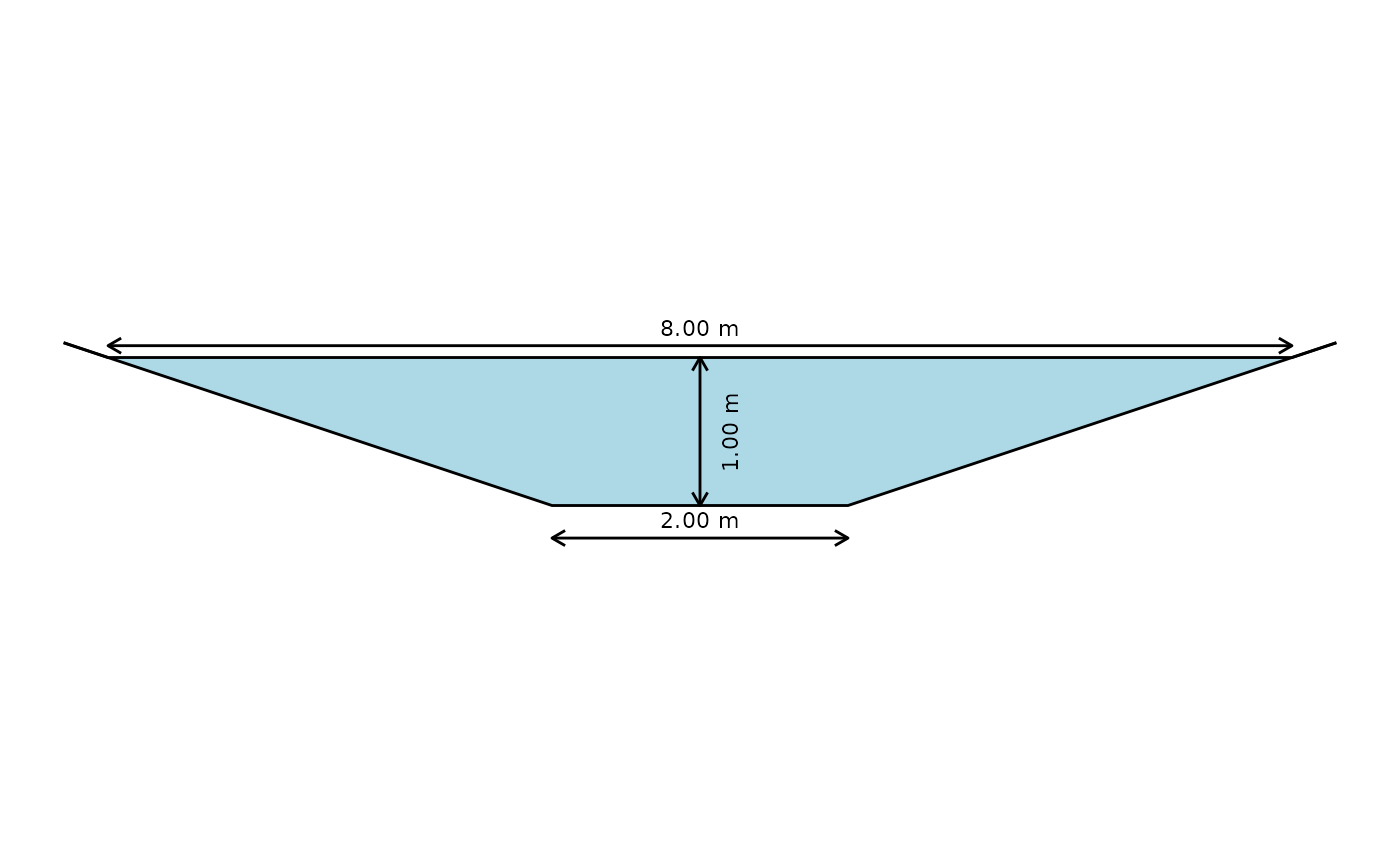This function plots a cross-section of a (trapezoid, rectangle, triangle), shaded as filled to the level indicated by the values passed to it.

## Usage

xc_trap(y = NULL, b = NULL, m = NULL, units = c("SI", "Eng"))

## Arguments

y

water depth [$$m$$ or $$ft$$]

b

bottom width [$$m$$ or $$ft$$]

m

side slope (H:1)

units

character vector that contains the system of units [options are SI for International System of Units and Eng for English (US customary) units.

## Value

a cross-section diagram

Ed Maurer

## Examples


# Draw a cross-section with depth 1, width 2, side slope 3:1 (H:V)
xc_trap(y = 1.0, b = 2.0, m = 3.0, units = "SI")